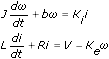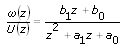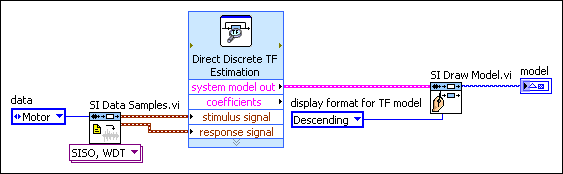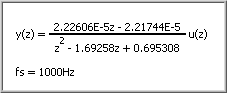# Identifying Discrete-Time Transfer Function Models (System Identification Toolkit)

LabVIEW 2013 System Identification Toolkit Help

Edition Date: June 2013

Part Number: 372458D-01

»View Product InfoDownload Help (Windows Only)

After physical modeling, you can describe the direct circuit (DC) servomotor by using the following differential equations:Set Ki to be equal to Ke to transform these differential equations. If you want to design a digital proportional-integral-derivative (PID) controller, transform these differential equations to the following continuous-time transfer function model:You can transform the continuous-time transfer function model to the following discrete-time transfer function model:where a0, a1, b0, and b1 are unknown parameters. ω is the rotational speed of the shaft. U is the driving voltage.

In this tutorial, you must estimate the values of a0, a1, b0, and b1 to identify the discrete-time transfer function model.

## Using the SI Transfer Function Estimation Express VI

Complete the following steps to identify the discrete-time transfer function model by using the SI Transfer Function Estimation Express VI.

1. Add the SI Transfer Function Estimation Express VI to the block diagram of the VI you created.

The Configure SI Transfer Function Estimation dialog box appears.
2. Select the Waveform button to identify dynamic models by using waveform input signals.
3. Click the Model Settings tab.
4. Select the Discrete button to identify discrete-time transfer function models.
5. Enter 1 in the Numerator order text box because the highest numerator order of this model is z.
6. Enter 2 in the Denominator order text box because the highest denominator order of this model is z2.
7. Click the OK button to close the configuration dialog box and return to the block diagram.
8. Wire the stimulus signal and response signal outputs of the SI Data Samples VI to the corresponding inputs of the SI Transfer Function Estimation Express VI.
9. Add the SI Draw Model VI to the block diagram.

10. Wire the system model out output of the SI Transfer Function Estimation Express VI to the system model input of the SI Draw Model VI.
11. (Optional) Create a constant for the display format for TF model input of the SI Draw Model VI and set the value to Descending.
12. Create an indicator for the model output of the SI Draw Model VI. The following figure shows the block diagram.13. Wire all error in and error out terminals on the block diagram.
14. Run this VI to identify the dynamic system model.

## Using the SI Estimate Transfer Function Model VI

Complete the following steps to identify the discrete-time transfer function model by using the SI Estimate Transfer Function Model VI.

1. Add the SI Estimate Transfer Function Model VI to the block diagram of the VI you created.

2. Click the polymorphic VI selector and select Discrete»Direct Method»Waveform.
3. Wire the stimulus signal and response signal outputs of the SI Data Samples VI to the corresponding inputs of the SI Estimate Transfer Function Model VI.
4. Create a control for the orders of transfer function model input of the SI Estimate Transfer Function Model VI.
5. Enter 1 in the num order field of this control because the highest numerator order of this model is z.
6. Enter 2 in the den order field of this control because the highest denominator order of this model is z2.
7. (Optional) Switch to the front panel, right-click this control, and select Data Operations»Make Current Value Default from the shortcut menu. LabVIEW uses the current value as the default value for this control.
8. Add the SI Draw Model VI to the block diagram.

9. Wire the system model out output of the SI Estimate Transfer Function Model VI to the system model input of the SI Draw Model VI.
10. (Optional) Create a constant for the display format for TF model input of the SI Draw Model VI and set the value to Descending.
11. Create an indicator for the model output of the SI Draw Model VI. The following figure shows the block diagram.12. Wire all error in and error out terminals on the block diagram.
13. Run this VI to identify the dynamic system model.

## Verifying Resulting Dynamic System Models

If you use the SI Data Samples VI to generate the input and output data in Part 2, LabVIEW returns the following system model:where a0=0.695308. a1=1.69258. b0=2.21744E–5. b1=2.22606E–5.